# Towards Reducing Repetitiveness in Community-Based Question Answering

Posted by Chi Zhang on November 26, 2018

This a summary of our Neurocomputing paper in solving the repetitiveness in Community-Based Question Answering.

## 1. Introduction

Community-Based Question Answering (CQA) platforms, such as Quora and Zhihu (Quora’s counterpart in China), are gaining enormous popularity among Internet users. Such service allows users to post questions that confuse them and provides an open area for those with specialized knowledge to share their insights on the questions. However, as the service coverage grows, large quantities of proposed questions become overlapped and redundant. This poses problems for new users, making it difficult for them to retrieve the best answers and worsening the situation by raising questions highly correlated with existing ones already with good answers.

In this work, we proposed a novel framework coined Heterogeneous Social Influential Network (HSIN) to reduce the repetitiveness in CQA platforms by effective information retrieval. We assume that by retrieving related questions already solved by other users, such algorithm would effectively prevent new users from asking repetitive questions, reducing the heavy burden from storage and retrieval and significantly improving the user experience in enjoying CQA service.

## 2. Rough Ideas in Attacking the Difficulties in CQA

#### 2.1. Semantics of Sentences and Sparsity of Words

One problem that makes the retrieval problem difficult is the semantic meaning of sentences. For example,

\begin{align*} & What\ are\ some\ good\ introductory\ materials\ on\ machine\ learning?\\ & How\ can\ I\ start\ learning\ machine\ learning? \end{align*}

Although these two sentences appear distinctive in the textual contents, their semantic meanings are similar.

Another difficulty comes from the sparsity of words, as these questions in such platforms are short in length. Both of these render the traditional feature engineering for natural languages, such as Bag-of-Words, less effective.

In our work, we leverage the state-of-the-art methods in language modeling to extract features for languages. Concretely, we use distributed representation for words, as introduced in my previous blog post, and the recurrent neural network to model questions.

#### 2.2. Information from Social Network

As could be inferred, CQA platforms are social networks in its nature. And one defining feature of the social network lies in its connectivity. Users are not only connected in CQA networks, but also linked with users in other service platforms, such as Twitter and Facebook. Though heterogeneous, such information embeds a huge amount of useful information for user profile modeling. And we believe this should also be helpful for user-specific question retrieval.

## 3. Retrieval via Heterogeneous Social Influential Network

In short, we utilize the questions’ textual content, their assigned categories, askers’ social information to simultaneously learn to rank the relativity of the queried question with those in the database.

#### 3.1. Problem Formulation

Before diving into the problem, let me briefly summarize the notation used in the blog post first. Given the textual representations of a set of questions $$X = \{x_1, x_2, ..., x_n\}$$, the corresponding features extracted are denoted $$Q = \{q_1, q_2, ..., q_n\}$$; the similarity of two questions $$q_i$$ and $$q_j$$ is measured by a metric function $$f_{q_i}(q_j) = q_i^Tq_j$$. And constraints from a set of triplets $$T = \{(j, i, k)\}$$ impose that

$f_{q_j}(q_i) \geq f_{q_k}(q_i)$

The set of triplets is obtained from a heterogeneous relation graph $$G = (V, E)$$, where $$V$$ contains nodes of users, questions and question tags. See Figure 1 for an illustrative example. We assume that questions post under the same tag or by two related users to have a higher similarity measure than others. Also, users have distributed representations denoted by $$e_w$$.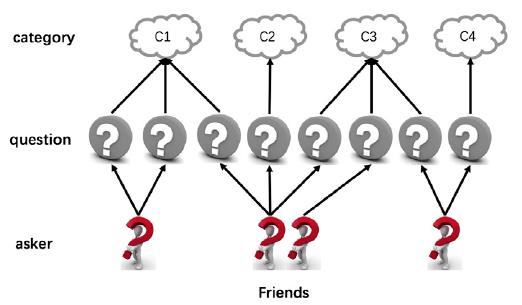Figure 1. Example of a heterogeneous graph where users are denoted as $$u$$, questions $$q$$ and tags $$c$$.

#### 3.2. Modeling Users and Questions

We borrow ideas from the DeepWalk model and apply a random walk sampler to our heterogeneous graph to generate random walk paths. Once paths are generated, they could be treated as word sequences and the word2vec model could be used to generate semantically meaningful embeddings for each node, including nodes for users. As for the questions, they are modeled by single-layer LSTM with each word represented as the off-the-shelf word embedding. Specifically, each word is encoded as its distributed representation and fed into the LSTM, which produces the final hidden state as the representation of the sequence.

These user embeddings and question embeddings are concatenated and further processed by several fully connected layers, which yield the final representation of the asker and question pair.

#### 3.4. Relative Rank Loss

To capture the nature of the retrieval problem, we design a loss function that better handles relative ranking of the question retrieval problem and we call it Relative Rank Loss:

$max\{0, m + f_{q_-}(q) - f_{q_+}(q)\}$

where $$m$$ is the margin, $$q_-$$ and $$q_+$$ are questions less and more related to $$q$$. Note that $$q$$ here is overloaded by its final representation combined with the user.

Putting all pieces together, the model is trained end-to-end.

The entire process is illustrated in Figure 2 and summarized in Algorithm 1.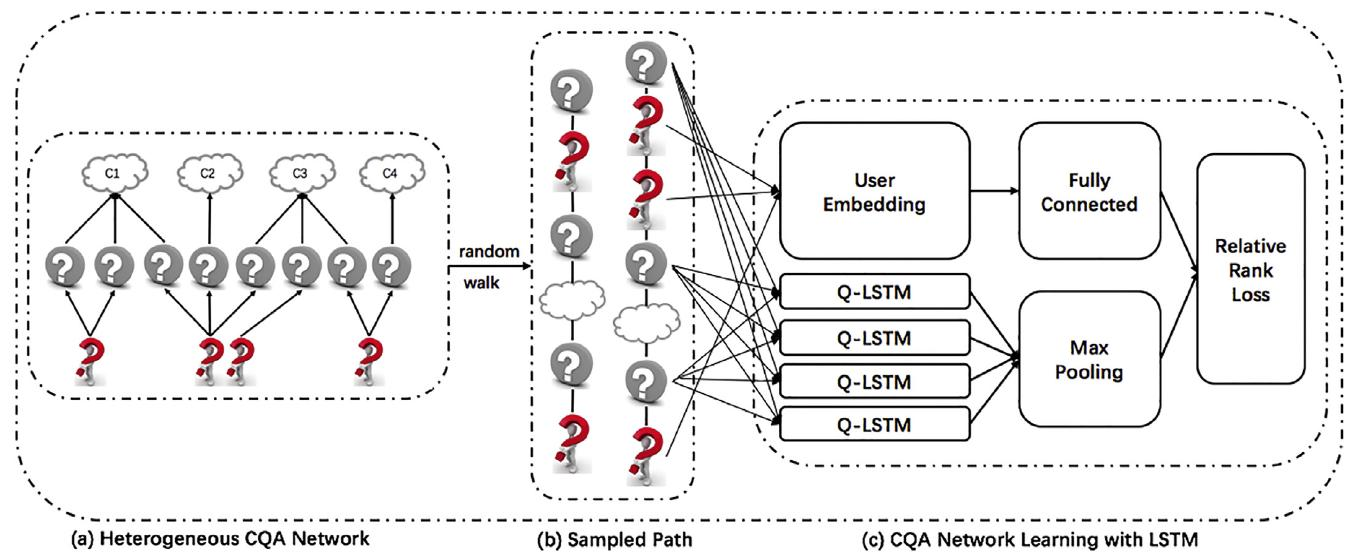Figure 2. The entire process of HSIN.Algorithm 1. The training algorithm of HSIN.

## 4. Performance

We evaluate the model’s performance on 4 metrics and observe significant improvement compared to 6 other solutions, verifying the effectiveness of our model.

Results are detailed as below.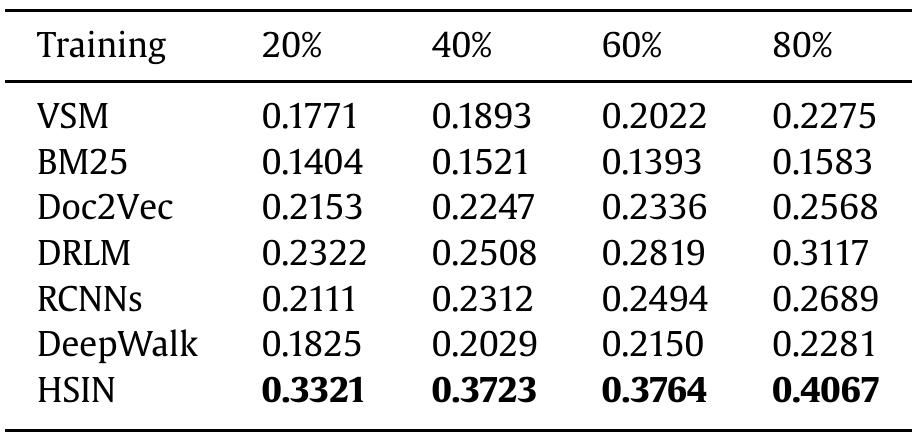Table 1. Results of MAP trained on different portions of data.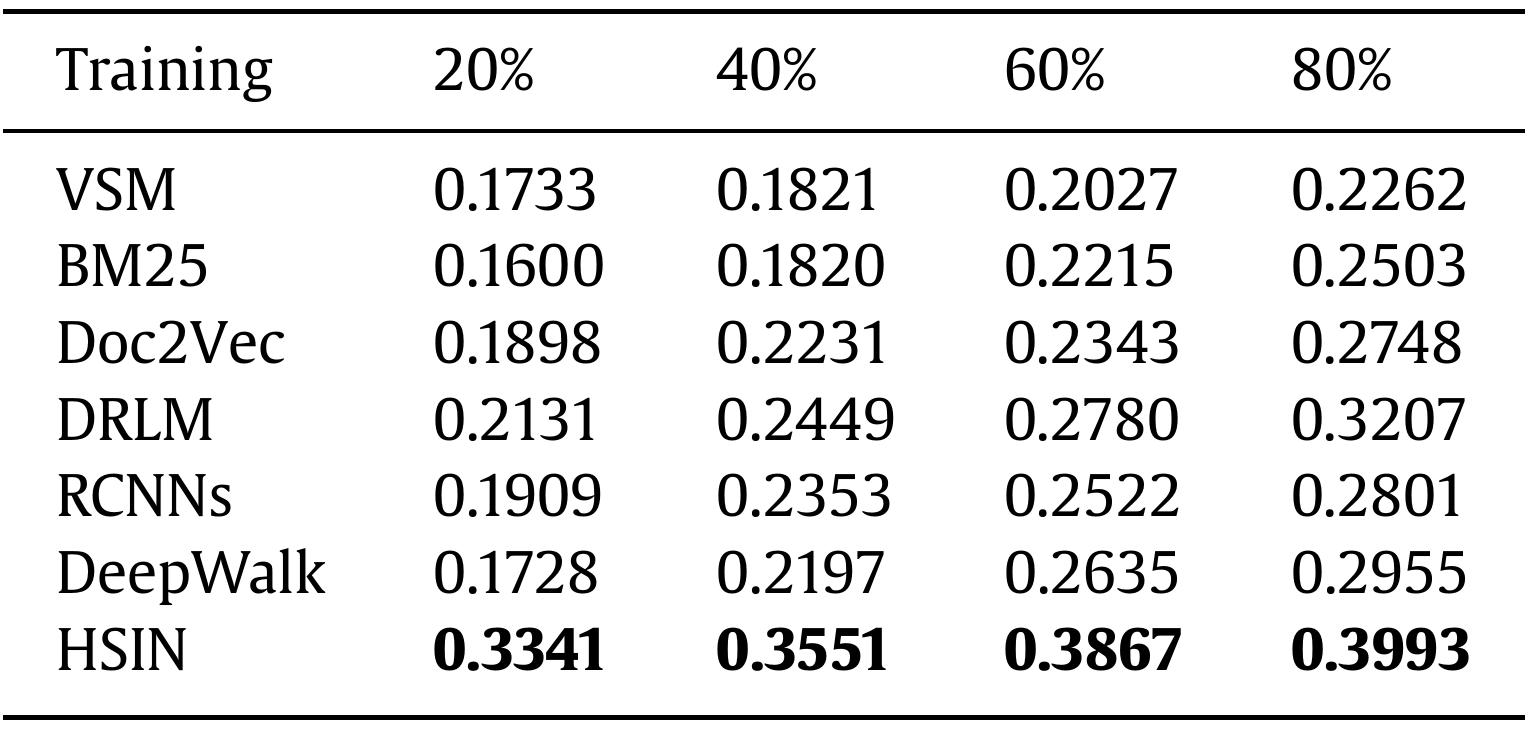Table 2. Results of Precision@1 trained on different portions of data.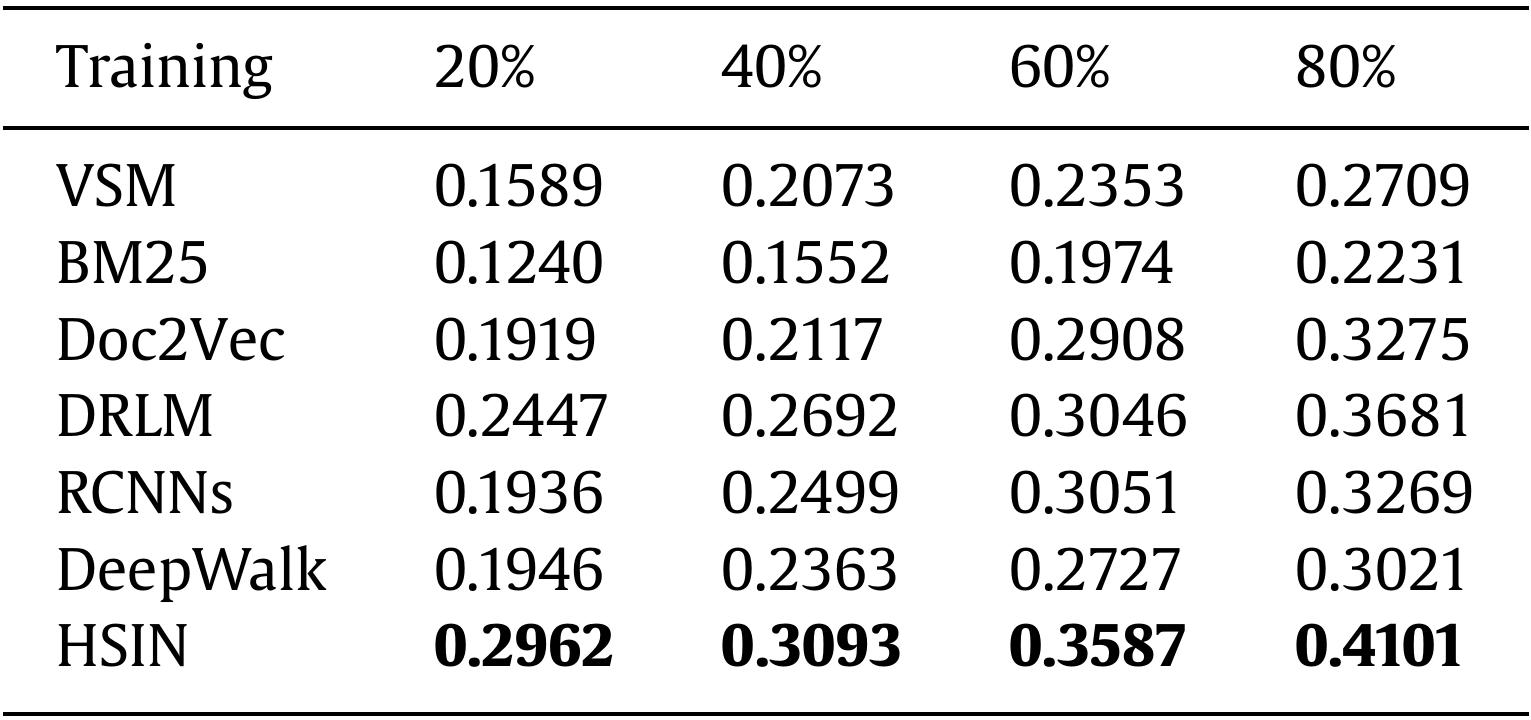Table 3. Results of Precision@5 trained on different portions of data.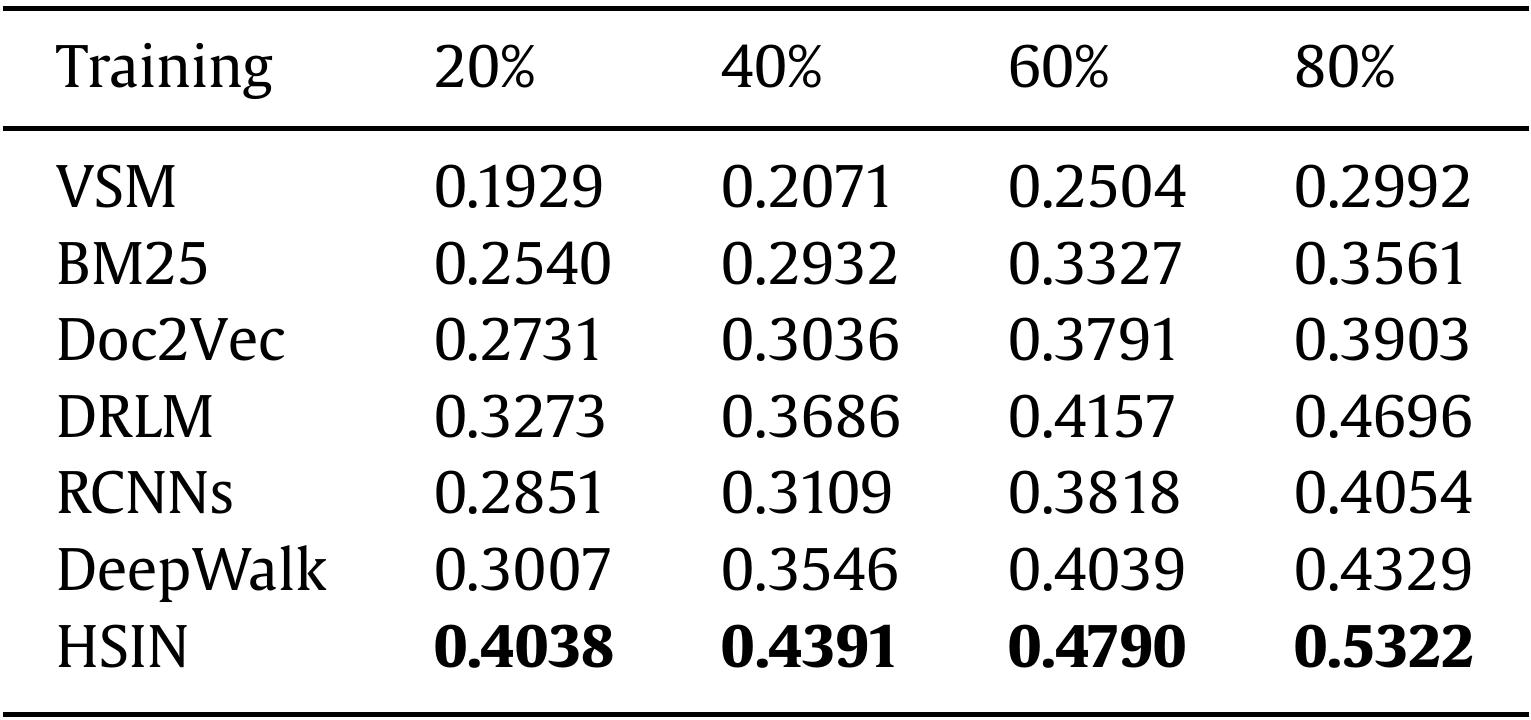Table 4. Results of MRR trained on different portions of data.

## 5. Discussion and Conclusion

Question retrieval is an essential component in CQA services. In this work we propose a new framework capable of exploiting category information associated with users’ social attributes to enhance the model’s ability in retrieval. We develop a random-walk-based method with recurrent neural network to measure question similarities in heterogeneous CQA networks. Experiments conducted on a large CQA data set demonstrates its superiority.

We believe that this work might open several interesting directions for future work. First, the idea of heterogeneous modeling could be applied to other fields in information retrieval. Second, detailed effects of related information from other networks deserves in-depth research. Finally, global relevance score and local relevance score might be further combined to boost the performance of retrieval model.

For details of the model and bibliography, please refer to our full paper.